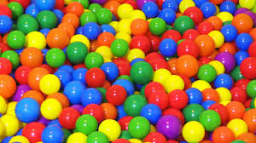# Busi has

Busi has a bag containing twenty balls. There are twice as many yellow balls than blue balls, but the yellow balls are only a third of the red balls. The green balls are the same number as the blue balls. How many are each of the colour ball?

y =  4
r =  12
b =  2
g =  2

### Step-by-step explanation:Did you find an error or inaccuracy? Feel free to write us. Thank you!

Tips for related online calculators
Do you have a linear equation or system of equations and looking for its solution? Or do you have a quadratic equation?